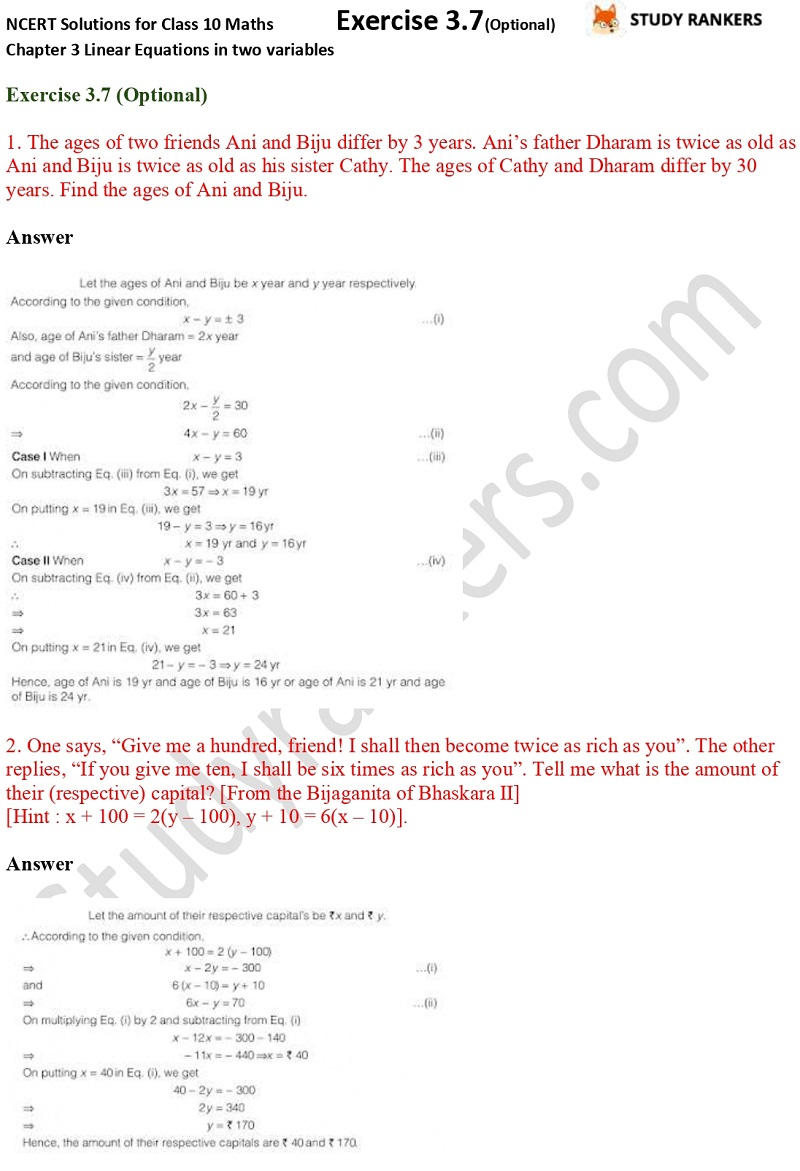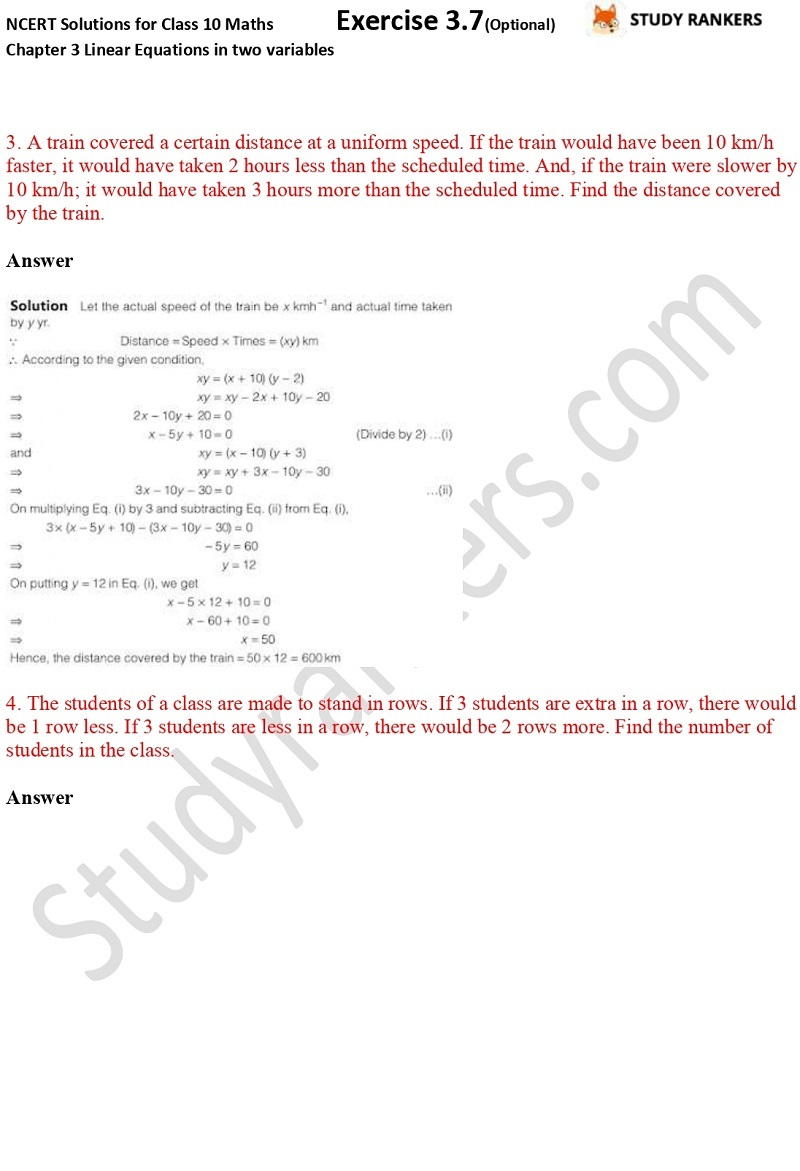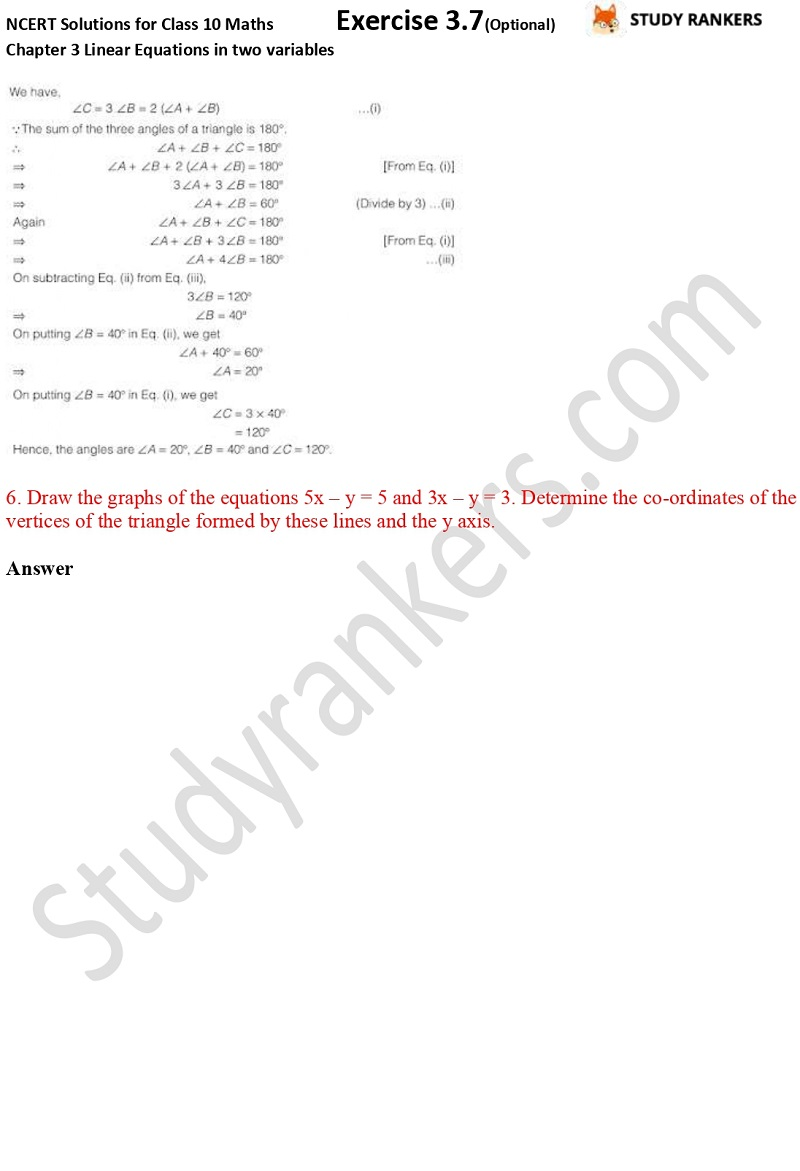## NCERT Solutions for Class 10 Maths Chapter 3 Pair of Linear Equations in Two Variables Exercise 3.7

In this page, you will find Chapter 3 Pair of Linear Equations in Two Variables NCERT Solutions for Class 10 Maths Exercise 3.7. These NCERT solutions are updated according to the latest NCERT Maths textbook. NCERT textbook are useful in building foundation and thus very much useful for Class 10 students to improve their marks. It will improve your problem solving skills and will introduce you to the basic concepts needed to solve questions.

There are total eight questions in this exercise where most of them are word problems such as The ages of two friends Ani and Biju differ by 3 years. Ani’s father Dharam is twice as old as Ani and Biju is twice as old as his sister Cathy. The ages of Cathy and Dharam differ by 30 years. Find the ages of Ani and Biju.